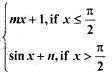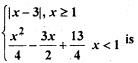## MCQ Quiz on Class 12 Maths Chapter 5 Continuity and Differentiability with Answers

We have compiled the MCQ Questions for Class 12 Maths Chapter 5 Continuity and Differentiability with Answers Pdf free download covering the entire syllabus for JEE and Boards. Practice MCQ Questions for Class 12 Maths with Answers on a daily basis and score well in exams. Refer to theContinuity and Differentiability Class 12 MCQs Quiz Questions with Answers here along with a detailed explanation

# Continuity and Differentiability Class 12 MCQs Questions with Answers

MCQ Questions for Class 12 Maths Chapter 5 Continuity and Differentiability with Answers

1. f (x) = max {x, x3},then the number of points where f (x) is not differentiable, are

2. If y = (1 + x)(1 + x2)(1 + x4)…..(1 + x2n), then the value of dy/dx at x = 0 is

3. Find dy/dx if x= a cos θ, y = b sin θ

4. For the function f(x) = x + 1/x, x ∈ [1, 3] the value of c for mean value theorem is

5. If f (x + y) = 2f (x) f (y) for all x, y ∈ R where f ‘ (0) = 3 and f (4) = 2, then f ‘ (4) is equal to

6. If y = (tan x)sin x, then dy/dx is equal to

7.  If f : R → R be a differentiable function, such that f (x + 2y) = f (x) + f (2y) + 4xy for all x, y ∈ R then

8. If f (x) = 2x and g (x) = x²/2 + 1, then’which of the following can be a discontinuous function

9. If x2 + y2 = 1, then

10. The derivative of y = (1 – x)(2 – x) ….. (n – x) at x = 1 is equal to

11.If f(x) =

is continuous at x = π/2, then

12. The set of points where the function f given by f (x) =| 2x – 1| sin x is differentiable is

13. Let function f (x) =14. If Rolle’s theorem holds for the function f(x) = x3 + bx2 + ax + 5 on [1, 3] with c = (2 + 1/3), find the value of a and b.

15. If sin y + e-xcos y = e, then dy/dx at (1, π) is equal to

16. The function f (x) = [x] is

17. Find dy/dx if x = cos3θ, y = sin3 θ

18. The function f(x) = e|x| is

19. The value of c in Rolle’s Theorem for the function f(x) = ex sin x, x ∈ [0, π] is

20. The number of discontinuous functions y(x) on [-2, 2] satisfying x2 + y2 = 4 is

Hope the information shed above regarding MCQ Questions for Class 12 Maths Chapter 5 Continuity and Differentiability with Answers Pdf free download has been useful to an extent. If you have any other queries of CBSE Class 12 Maths Continuity and Differentiability MCQs Multiple Choice Questions with Answers, feel free to comment below so that we can revert back to us at the earliest possible.
By Team Study Rate

### Sneha

Master's in Biology, Skilled in vocational training. Strong Analytical and creative knowledge.

This site uses Akismet to reduce spam. Learn how your comment data is processed.